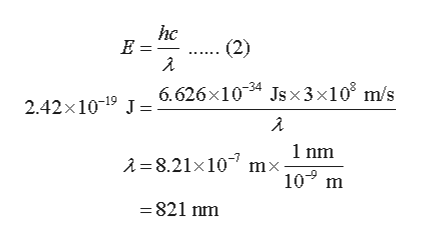# Calculate the maximum wavelength of light capable of removing an electron for a hydrogen atom from the energy state characterized by the following.n = 3n = 4

Question
2 views

Calculate the maximum wavelength of light capable of removing an electron for a hydrogen atom from the energy state characterized by the following.
n = 3

n = 4

check_circle

Step 1

The energy of a state (E) in the hydrogen atom is calculated using equation (1) in which n = 1, 2, 3 ……

Step 2

The energy of an electron for n = 3 (E3) is calculated using equation (1) as follows:

Step 3

The energy of the electron present in n = 4 is – 2.42×10-19 J. The energy of light required to remove this electron i...help_outlineImage Transcriptionclosehc (2) 6.626x1034 Jsx3x108 m/s 2.42x1019 J 1 nm A=8.21x10 mx 10 m =821 nm fullscreen

### Want to see the full answer?

See Solution

#### Want to see this answer and more?

Solutions are written by subject experts who are available 24/7. Questions are typically answered within 1 hour.*

See Solution
*Response times may vary by subject and question.
Tagged in

### Chemistry